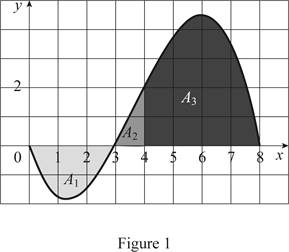# The following integral in the increasing order. (A) ∫ 0 8 f ( x ) d x (B) ∫ 0 3 f ( x ) d x (C) ∫ 3 8 f ( x ) d x (D) ∫ 4 8 f ( x ) d x (E) f ′ ( 1 )### Single Variable Calculus: Concepts...

4th Edition
James Stewart
Publisher: Cengage Learning
ISBN: 9781337687805### Single Variable Calculus: Concepts...

4th Edition
James Stewart
Publisher: Cengage Learning
ISBN: 9781337687805

#### Solutions

Chapter 5.2, Problem 47E
To determine

## To list: The following integral in the increasing order.(A) ∫08f(x) dx(B) ∫03f(x) dx(C) ∫38f(x) dx(D) ∫48f(x) dx(E) f′(1)

Expert Solution

The increasing order of the integral function is B<E<A<D<C_.

### Explanation of Solution

Given:

The graph of the function f is shown in Figure 1.

Calculation:

Plot the graph of the function f as shown below:Refer the figure 1.

Consider the area under the graph are A1, A2, and A3.

The area A1 is negative as it is below the x-axis.

The area A1 is equal to integral 03f(x)dx.

The area A2 is equal to integral 34f(x)dx.

The area A3 is equal to integral 48f(x)dx.

The first smallest value among the integral is 03f(x)dx.

The area denoted by 03f(x)dx is the maximum negative area under the function f.

The second smallest area under the plot is f(1).

The value of f(1) is the value of the tangent at x=1.

The value of f(1) will be between 0 and 1.

The third smallest area under the plot is 08f(x)dx.

This is the difference of maximum positive area and maximum negative area.

The largest area under the plot is 38f(x)dx.

The area denoted by 38f(x)dx is the maximum negative area under the function f.

The second largest area under the plot is 34f(x)dx.

Refer to the Figure 1.

38f(x)dx=A2+A3=34f(x)dx+48f(x)dx48f(x)dx=38f(x)dx34f(x)dx

Thus, the area denoted by 34f(x)dx is less than the area 38f(x)dx.

Show the area in the increasing order as follows:

03f(x)dx<f(1)<08f(x)dx<48f(x)dx<38f(x)dxB<E<A<D<C

Therefore, the increasing order of integral is B<E<A<D<C_.

### Have a homework question?

Subscribe to bartleby learn! Ask subject matter experts 30 homework questions each month. Plus, you’ll have access to millions of step-by-step textbook answers!